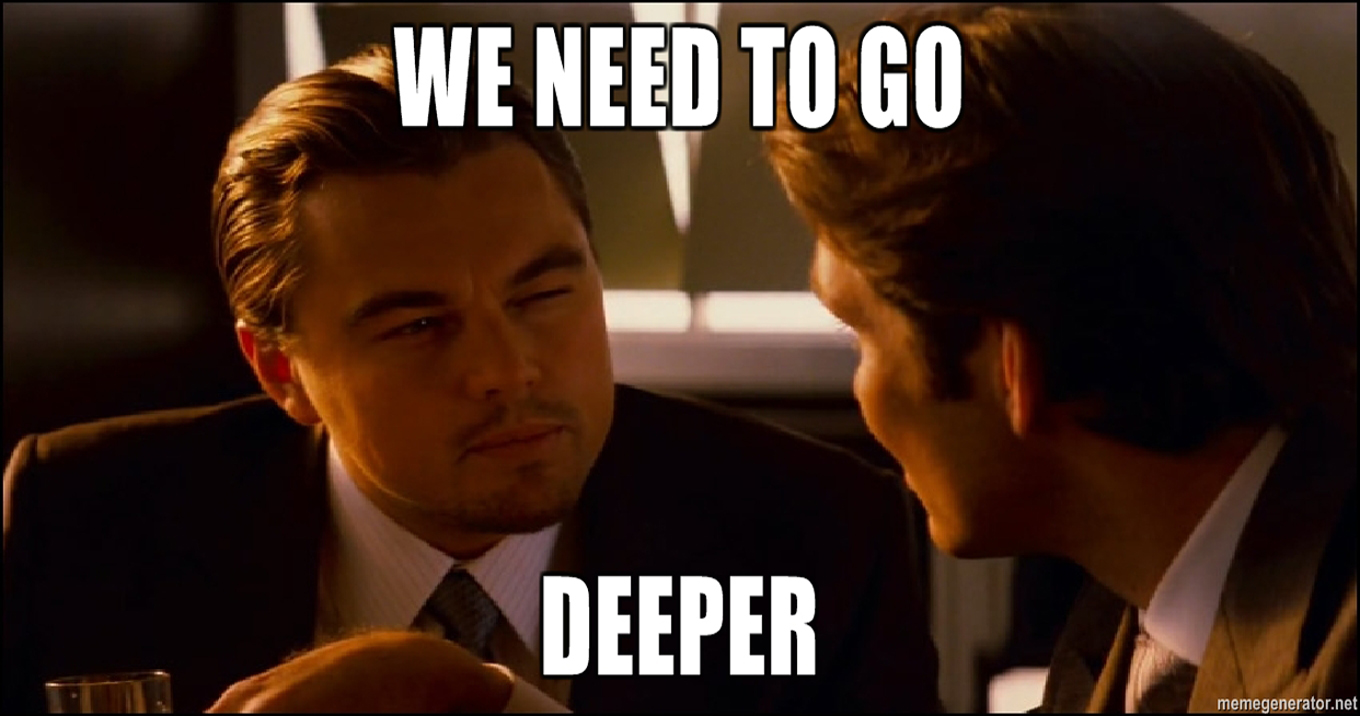## Welcome to the Treehouse Community

Want to collaborate on code errors? Have bugs you need feedback on? Looking for an extra set of eyes on your latest project? Get support with fellow developers, designers, and programmers of all backgrounds and skill levels here with the Treehouse Community!

### Looking to learn something new?

Treehouse offers a seven day free trial for new students. Get access to thousands of hours of content and join thousands of Treehouse students and alumni in the community today.# Is the code that used for this challenge "correct?"

```function genNumber(high,low) {
return Math.floor(Math.random() * (high - low+1)) + low;
}

document.write(genNumber(parseInt(prompt('Give me a high number')), parseInt(prompt('Give me a low number'))))
```

Please see the document.write statement that I used to pass the argument to the function, and simultaneously write the return.

It worked fine for me, but would it be better to use a longer way that is easier to understand (and perhaps less likely to contain errors?)

it all depends on the coding style and what you are comfortable with. if you are working in a team then you might write it in an easier to read style..I have noticed that using your function with say a range of 22 to 23, a person will never get 22. In fact, the lower region does not appear, so it does not give a uniform distribution.

So, it is actually not truly random in the sense that all probabilities are given equal chance to appear, which means that some numbers will appear at a higher frequency than others, aka the lower limit never appearing.Thank you for pointing that out Anthony. I have revised the code below to account for the user error of choosing a lower 'high' number and a higher 'low' number.

```function genNumber(high,low) {
return Math.floor(Math.random() * (high - low+1)) + low;
}

var high = parseInt(prompt('Give me a positive integer GREATER than 1'));
var low = parseInt(prompt('Give me a positive integer LESSER than the first one'))

while (low >= high || high<1) {
var high = parseInt(prompt('Give me a positive integer GREATER than 1'));
var low = parseInt(prompt('Give me a positive integer LESSER than the first one'));
}

document.write (genNumber(high,low));
``````/*
Great code. I have a small edit though.

How about just having all the code related to the function
in the function itself.

That way you keep all the actions local
to the function you are working with
and avoid global variables.

It keeps things nice and modular.
- AA
*/

function genNumber(high,low) {

//get values
high = parseInt(prompt('Give me a positive integer GREATER than 1'));
low = parseInt(prompt('Give me a positive integer LESSER than the first one'));

// sort out to include higher or lower
// great way to address problem by the way.
while (low >= high || high<1) {
high = parseInt(prompt('Give me a positive integer GREATER than 1'));
low = parseInt(prompt('Give me a positive integer LESSER than the first one'));
}

return Math.floor(Math.random() * (high - low+1)) + low;
}

document.write(genNumber(high,low));
```I used to think that if I could jam several lines of code into a single line of strategically nested inception functions, it was somehow more "elegant".

In hindsight, after revisiting my code months later (or worse: debugging my code months later), I realized what a pain it is to have to mentally unpack dense code. Even if what would have been 20 lines was neatly condensed to 5, the extra step of having to dissect and parse out code as you read prevents any sort of flow when you may just be trying to skim the program.If you're just starting out, it's probably best to keep syntax as simple and as logical as possible. This has definitely helped me out as I continue to learn how condense code more pragmatically (i.e., using functions and arrays and loops, etc.) instead of just condensing economically to spare a few variables or lines of code.